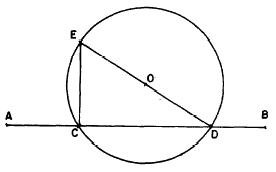Perpendicular at any point on a lineCustom SearchPERPENDICULAR AT ANY POINT ON A LINE Figure 18-4 shows a line AB with point C between A and B. A perpendicular to AB is erected at C as follows:Figure 18-3.-Bisecting a line geometrically.Figure 18-4.-Erecting a perpendicular at a point. 1. Using any convenient point above the line (such as 0) as a center, draw a circle with radius OC. This circle cuts AB at C and at D. 2. Draw line DO and extend it to intersect the circle at E. 3. Draw line EC. This line is perpendicular to AB at C. BISECTING AN ANGLE Let angle AOB in figure 18-5 be an angle which is to be bisected. Using 0 as a center and any convenient radius, draw an arc intersecting OA and a second arc intersecting OB. Label these intersections C and D. Using C and D as centers, and any convenient radius, draw two arcs intersecting halfway between lines OA and OB. A line from 0 through the intersection of these two arcs is the bisector of angle AOB.Figure 18-5.-Bisecting an angle. SPECIAL ANGLES Several special angles may be constructed by geometric methods, so that an instrument for measuring angles is not necessary in these special cases. Figure 18-4 illustrates a method of constructing a right angle, DCE, by inscribing a right triangle in a semicircle. But an alternate method is needed for those situations in which drawing circles is inconvenient. The method described herein makes use of a right triangle having its sides in the ratio of 3 to 4 to 5. -It is often used in laying out the foundations of buildings. The procedure is as follows: 1. A string is stretched as shown in figure 18-6, forming line AC. The length of AC is 3 feet. 2. A second string is stretched, crossing line AC at A, directly above the point intended as the corner of the foundation. Point D on this line is 4 feet from A. 3. Attach a third string, 5 feet long, at C and D. When AC and AD are spread so that line CD is taut, angle DAC is a right angle. A 60° angle is constructed as shown in figure 18-7. With AB as a radius and A and B as centers, draw arcs intersecting at C. When A and B are connected to C by straight lines, all three angles of triangle ABC are 60° angles. The special angles already discussed are used in constructing 45° and 30° angles. A 90° angle is bisected to form two 45° angles, and a 60° angle is bisected to form two 30° angles.Integrated Publishing, Inc. - A (SDVOSB) Service Disabled Veteran Owned Small Business